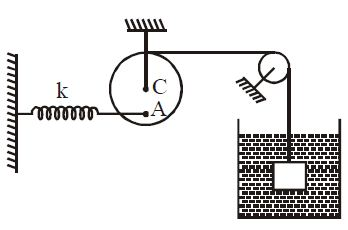# Find the Compression -2!A cubical block of volume $V$ and density $3\rho$ is placed inside a liquid of density $\rho$ and attached to a spring of spring constant $k$ as shown in the figure. Assuming ideal spring and pulley and spring is attached at $A$ which is at $\frac{R}{2}$ from center. The compression in the spring at equilibrium is:

×